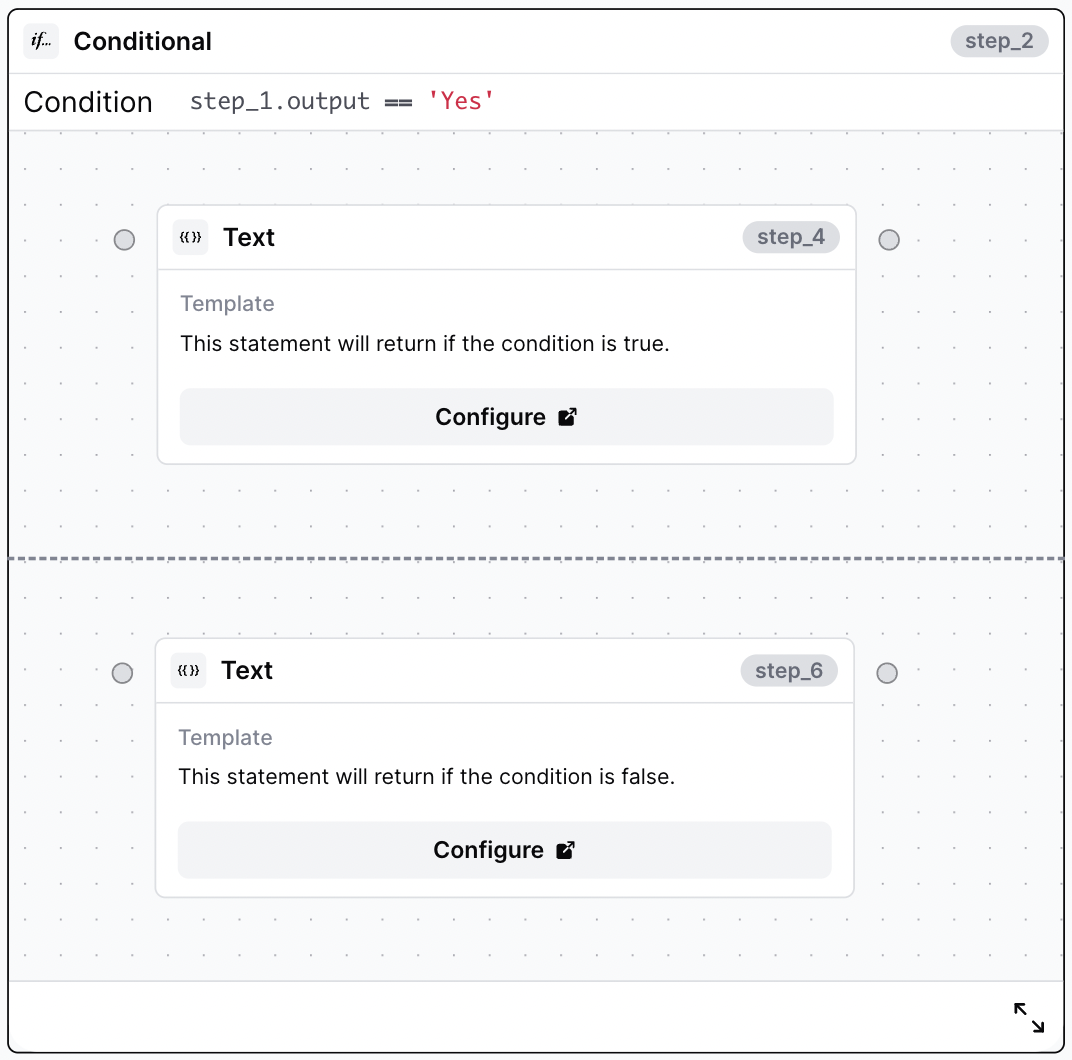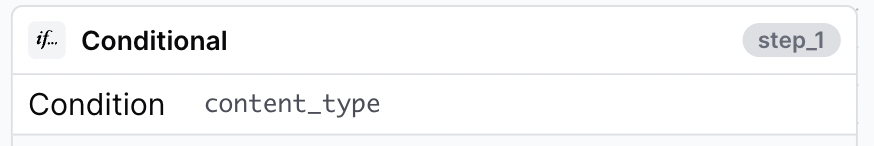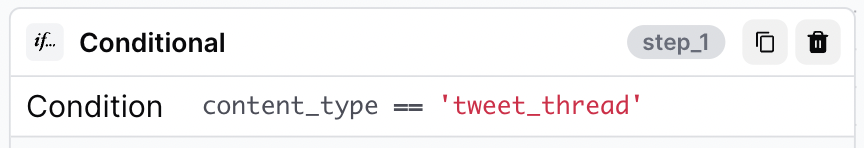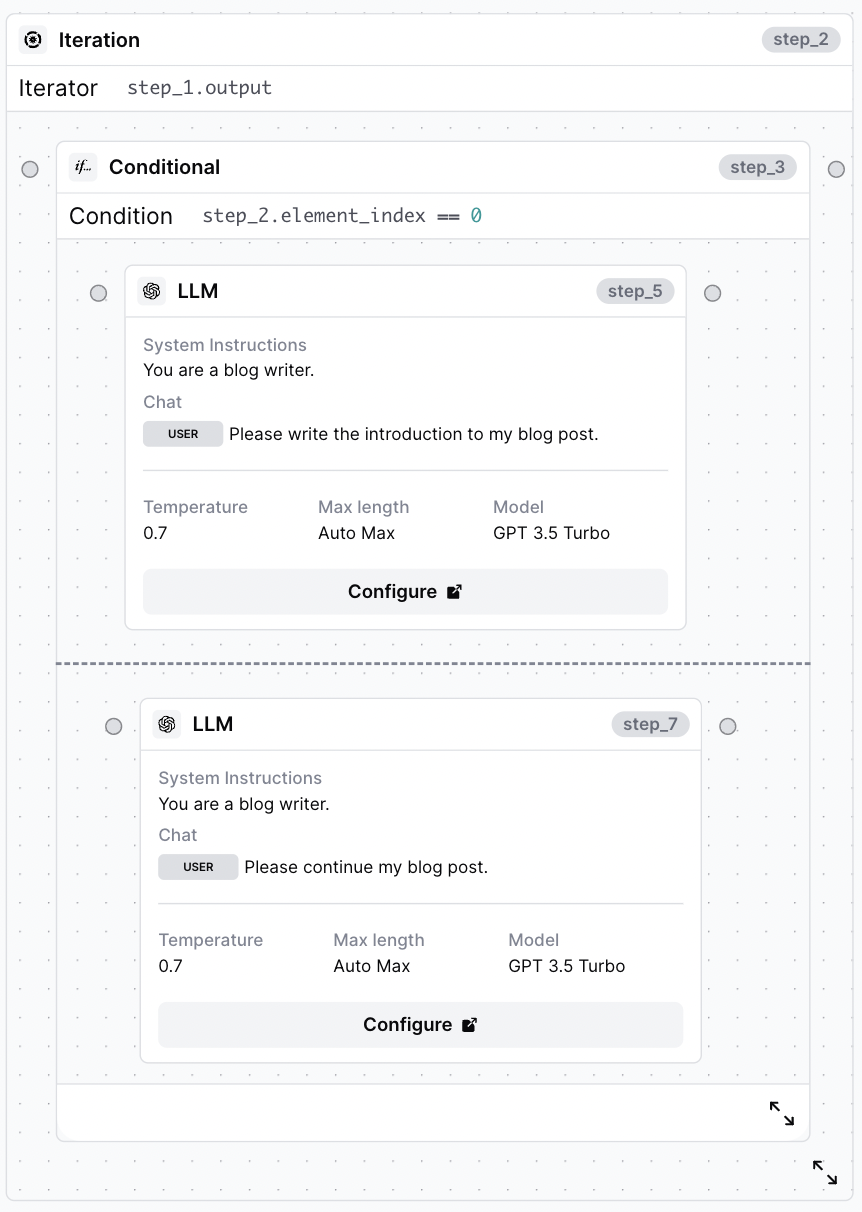# Conditional Step

Branch your workflow logic with a conditional step

A conditional step allows you to generate outcomes based on a specific condition you set, similar to an if-else statement.

### Configuration

The `condition` is a Javascript statement that should evaluate to `true` or `false` .

If `condition` evaluates to `true`, the steps in the top half of the conditional will be executed. If `condition` evaluates to `false`, the steps in the lower half of the conditional will be executed.

For example, if `step_1.output == 'Yes'` evaluates to `true`, `step_4` will be executed; otherwise, `step_6` will be executed:### Conditional Examples

Let's take a look at how to set up the conditional with the following examples:

#### Check if the user passed an optional input

Assume we have an optional input field called `content_type`. We can check if the user submitted a value to the optional field by passing `content_type` as the condition:#### Apply different logic based on the value of a drop-down selection

Now, let's assume `content_type` is a dropdown with multiple options. We can change the output depending on the option that is selected. For example, if a user selects `tweet_thread`, we can write an LLM step to generate a tweet thread; otherwise, we can generate a different type of social, like a LinkedIn post.#### Write a blog with using different logic for the introduction

To write the first part of a blog differently than the rest of a blog, we would:

1. Iterate over all sections of a blog with an iteration step
2. Use a conditional step to apply different logic to the first iteration. In the example below, `step_2.element_index == 0` means execute the top LLM step of the first iteration; otherwise, execute the bottom LLM step for every subsequent iteration.
3. Write the prompt for the introduction of the blog in the top half of the conditional
4. Write the prompt for the rest of the blog in the bottom half of the conditional